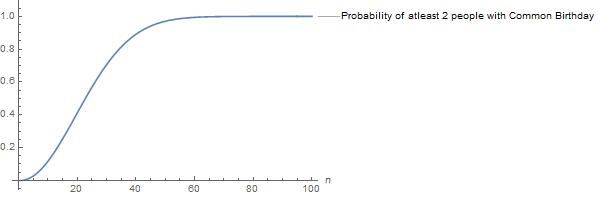top of page
Search
• Ganesh Balasubramanian

# The shared birthday problem!

Updated: Oct 27, 2021

Imagine you are part of team, what are the chances that no two people in your team will share the same birth date? The numerical implications of some probabilities are exciting. For example, if there are more than 23 people in your team/office, then there is more than 50% chance that at least two among the 23 will have a joint birthday. If this statement is intriguing, then you probably will enjoy the reading this article.Imagine there are 'n' people in a room. Let us find the probability that no two people in the room will share a common birth date.

To make the math simple, let us assume that there are 365 days in a year (we ignore the leap years).

Let every day of the year be uniquely represented by numbers from the set {1,2,3…,365}

So the birthday of a person will correspond to a particular number from the set {1,2,3…,365}.

If there are no joint birthdays, for n people in the room, we will have n different numbers representing their respective birth dates. So, we find the number ways in which we can select n different numbers from the set {1,2,…,365}. No: of ways of selecting n different numbers from 365 numbers is given by 365 * 364 * ….. *365-(n-1). The first number can be selected in 365 ways the second number in 364 ways (all except the previously selected number since repetition is not allowed as there should be no common birthdays) so on and so forth.

Next, we find the total number of ways in which any n numbers can be selected from the set {1,2,…365}. This is given by 365*365*…n times = 365^n. The first number can be selected in 365 ways the second number can also be selected in 365 ways so on and so forth. (Remember here there is no condition that numbers should not be repeated)

The probability that there are no joint birthdays for the n people in the room is

p=(365*364*....*(365-(n-1))) / (365^n)

The above result has an exciting numerical implication. We can see that if n is greater than 23 then P<0.5. This implies that if there are more than 23 people in a room, then the probability that at least two people have common birthday is more than half. Similarly, in a room with more than 50 people, the probability that at least 2 people share the same birthday is greater than 90%. The below plot illustrates the probability of at least 2 people sharing the same birth date against the number of people ('n') in the group.References

Feller, W. (2008). An introduction to probability theory and its applications (Vol. 2). John Wiley & Sons.